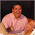## Tuesday, 16 February 2016

1.simple quadratic equation. Bunny = 4Kg, Cat = 6Kg, Dog = 18 Kg

1.If bunny was 4 and dog was 18 then bunny plus dog would be 22, not 20. They were each weighed twice, add up each weighing and divide by two and the total is 27. It doesn't matter what each weighs separately.

2.3.4.I'd rather figure out how all the animals are able to read the scale when there are two of them on it but not when there are three. And I would LOVE to know how they are able to speak!

5.It's 27 kg. If we sum up the first three pictures, we get 54 kg, and that would have two bunnies, two cats, and two dogs. Divide by two and you get their combined 27 kg.

6.27kg. 3, 7, and 17kg for the bunny, cat, and dog.

Quadratic equations do not work for this. You can model it as a system of linear equations.
1b + 1c + 0d = 10
1b + 0c + 1d = 20
0b + 1c + 1d = 24

You can do some substitutions and figure out out what one of the variables is and the rest fall through. Or... you can turn it into a matrix math problem.

http://www.bluebit.gr/matrix-calculator/linear_equations.aspx

A is:
1 1 0
1 0 1
0 1 1

B is:
10
20
24

https://www.mathsisfun.com/algebra/systems-linear-equations-matrices.html

The mechanics of matrix math are a little overwhelming the first time you see it. That's OK because humans really don't solve matrix equations all that often, computers do. There are a quite a few more steps and numbers involved, but those steps are the same for any linear equation and computers are good at handling lots of numbers. So, if you can translate a problem into a matrix math (linear algebra) problem, then you can let a computer do the work.

OK. Let's solve it the way a meat-bag would solve it. Just for fun.

1b + 1c + 0d = 10 [equation X]
1b + 0c + 1d = 20 [equation Y]
0b + 1c + 1d = 24 [equation Z]

So, take take equation X and subtract equation Z from it.

1b + 0c - 1d = -14 [equation X']

We can do this because we can add/subtract any amount we want, so long as we do it from both sides. Since we know the left and right half of equation Z are equal, we can subtract the symbols from the left, and the numbers from the right.

This (equation X') is nice since we now have two different equations without cats in it (Y and X'). Let's add equation Y to it to get rid of dogs too.
2b + 0c + 0d = 6

Now all we have are bunnies and weights. Solve for b, and you get b=3. You can now figure out the rest pretty easily. Cat is 7 (via equation X) and Dog is 17 (via equation Y).

OK, and another trick because I apparently have too much time on my hands...
1b + 1c + 0d = 10 [equation X]
1b + 0c + 1d = 20 [equation Y]
0b + 1c + 1d = 24 [equation Z]

2b + 2c + 2d = 54

Divide by 2.
1b + 1c + 1d = 27

7.(bunny + cat) + (bunny + dog) + (cat + dog) = 10 + 20 + 24, add everything
2bunny + 2cat + 2dog = 54, divide by 2
bunny + cat + dog = 27

8.r+c=10
r+d=20
c+d=24

2r+c+d=30
c+d=24
(subtract the bottom line from the top)
2r=8
r=4

plug r=4 into the top equations to get
c=6
d=18

All together:
c+r+d = 28

1.On line 7 you made the mistake of 30 - 24 = 8 when it should be 6.
This then in turns gives you;
r = 4 instead of 3,
c = 6 instead of 7,
d = 18 instead of 17, and
r+c+d = 28 instead of 27.

2.Argh, sorry, typo. That's what you get for doing mental arithmetic in bed.

2r+c+d=30
c+d=24
(subtract the bottom line from the top)
2r=6
r=3

plug r=3 into the top equations to get
c = 7
d = 17

All together:
c+r+d = 27

3.'cept for the bit where 2r should be 6 when you did your subtraction. (30-24 = 6)

9.Bunny 3kg cat 7kg dog 17 kg equals 27kg

10.(1) R + C = 10;
(2) R + D = 20;
(3) C + D = 24;
(4) R + C + D = ?

(5) From equations (1) and (2) we can subtract R to tell us that the difference between C and D must be 10.

(6) From equations (2) and (3) we can subtract D to tell us that the difference between R and C must be 4.

(7) Since we know that R + C = 10, we can substitute our deduction from (6), R + (R + 4) = 10, 2R + 4 = 10, R = 3

(8) Since we now know R, we can substitute it any either of the other equations to get the values of C and D;
(1); 3 + C = 10, C = 7
(2); 3 + D = 20, D = 17;

Therefore: (4): R + C + D = 3 + 7 + 17 = 27.

11.An no Rachel, it's not a quadractic equation, there are no exponents involved whatsoever.

12.talking animals!

13.You lot are so smart! Hey wait...did someone say TALKING ANIMALS??? ;)

14.The scale is broken. The arrow doesn't move for the first three weighings, then when they all get on, it displays a question mark.